>

## NCERT Solutions for Class 11 Maths Chapter 13 Limits and Derivatives Exercise 13.2

Chapter 13 Limits and Derivatives Exercise 13.2 NCERT Solutions for Class 11 Maths will help you in solving your doubts as these Class 11 Maths NCERT Solutions are prepared by Studyrankers experienced subject matter experts. These NCERT Solutions are very important for the purpose of examinations as it will help you knowing the basic concepts of the chapter.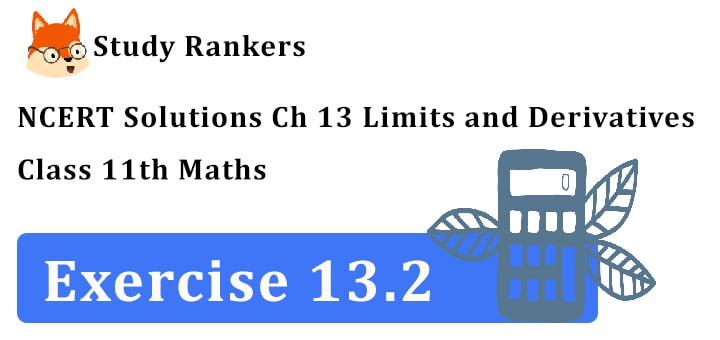1. Find the derivative of x 2 – 2 at x = 10

Let y = x 2 – 2
dy/dx = 2x dy/dx at x = 10 is equal to 20

2. Find the derivative of x 2 – 2 at x = 10
Find the derivative of 99x at x = 100 (By first principle)

Let f (x) = 99x. Derivative of f (x) at x = 100 isNow, f (x) = 99x
f (100 + h)= 99(100 + h)
f (100)= 99 × 100
∴ f (100 + h) – f (100) = 99 (100 + h) – 99 × 100
= 99 [100 + h – 100] = 99 × h3. Find the derivative of x at x = 1

Derivative of f (x) = x at x = 14. Find the derivative of the following functions from first principle:
(i) x 3 – 27 (ii) (x – 1)(x – 2)
(iii) 1/x2 (iv) (x + 1)/(x – 1)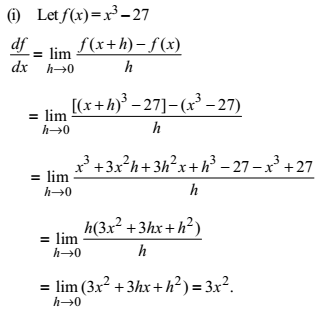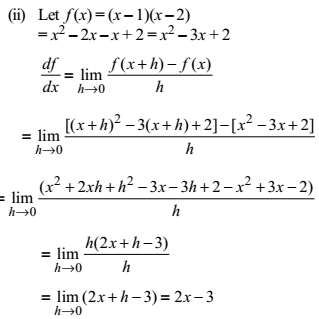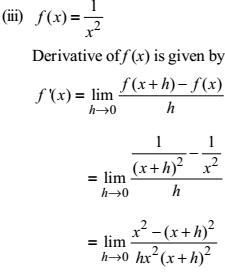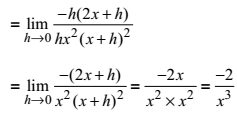5. For the function
f(x) = x100/100 + x99/99 + ……..+ x2/2 + x + 1
prove that f’(1) = 100 f’(0)

We know that d/dx (xn) = nxn-1
∴ For f(x) = x100/100 + x99/99 + ……+ x2/2 + x+ 1
f'(x) = 100x99/100 + 99. x98/99 + …..+ 2x/2 + 1
= x99 + x98 + ………….+ x + 1
Now, f’(x) = 1 + 1+ …….. to 100 term = 100
f'(0) = 1
∴ f'(1) = 100 × 1 = 100f’(0)
hence, f’(1) = 100f’(0)

6. Find the derivative of x n + axn – 1 + a 2n – 2 + ....+ a n – 1x + a n for some fixed real number a?

Let f (x) = xn + axn – 1 + a 2 x n – 2+ ........ + a n – 1x + a n
Now, d/dx xn = nxn-1 , d/dx xn-1 = (n – 1)xn-2.
etc and d/dx [ag(x)] = ag’(x), d/dx an = 0
f’(x) = nxn-1 + (n – 1)axn – 2 + (n – 2)a2xn-3 + ………..+ an-1

7. For some constants a and b, find the derivative of:
(i) (x – a)(x – b)
(ii) For some constant a and b find the derivation of (ax2 + b) 2 .
(iii) (x – a)/( x – b)

(i) Let f (x) = (x – a)(x – b)
Using product rule, we have
df(x)/dx = (x – a) d(x – b)/dx + (x-b) d(x-a)/dx
= (x – a)[d(x)/dx - d(b)/dx] + (x – b)[d(x)/dx - d(a)/dx]
= (x – a)[1 – 0] + (x – b)[1 – 0]
(ii) f(x) = (ax2 + b)2 = a2x+ 2abx2 + b2
now, d/dx x4 = 4x3 and
d/dx x2 = 2x, d/dx b2 = 0
f’(x) = a2. 4x3 + 2ab.2x + 0
= 4a2x3 + 4abx
(iii) Let f(x) = (x- a)/(x – b)
Using quotient rule, we have
= ((x – b)(d(x-a))/dx – (x – a) (d(x – b))/dx)/(x – b)28.  Find the derivative of (xn – an)/(x – a) for some constant ‘a’.

We know d/dx (u/v) = (u’v – uv’)/v2
d/dx ((xn – an)/(x – a))
= ([d/dx (xn – an)](x – a)-(xn – an)d/dx (x – a))/(x – a)2
= (nxn-1(x – a)- (xn – an). 1)/(x – a)2
= (nxn – n.xn – 1 a – xn + an)/(x – a)2
= ((n – 1 )xn – naxn-1 + an)/(x – a)2

9. Find the derivative of
(i) 2x – 3/4
(ii) (5x3 + 3x – 1)(x – 1)
(iii) x-3(5 + 3x)
(iv) x5(3 – 6x-9)
(v) x-4(3 – 4x-5)
(vi) 2/(x + 1) - x2/(3x – 1)

(i) Let f(x) = 2x – 3/4
f'(x) = 2 (d/dx) x = 1, (d/dx)(3/4) = 0

(ii) let f(x) = (5x3 + 3x – 1)(x – 1)
(uv)’ = u’v + uv’
f'[(5x3 + 3x – 1)(x – 1)]
= [d/dx (5x3 + 3x – 1)](x – 1) + (5x3 + 3x – 1) d/dx (x – 1)
= (15x2 + 3)(x – 1) + (15x3 + 3x – 1).1
= x(15x2 + 3) – (15x2 + 3) + (5x3 + 3x – 1))
= 20x3 – 15x2 + 6x - 4

(iii) let f(x) = x-3 (5 + 3x) = 5x-3 + 3x-2
f’(x) = 5(-3)x-4 + 3(-2)x-3
= (-15/x4) – (6/x3) = (-(6x + 15))/x4 = (-3(2x + 5))/x4
(iv) Let f(x) = x5(3 – 6x-9) = 3x5 – 6x-4
f'(x) = (d(3x5 – 6x-4))/dx
= (d(3x5)/dx) – (d(6x-4)/dx)
= 3.(dx5/dx) – 6.(dx-4/dx)
= (3)(5)x4 – (6)(-4)x-5
= 15x4 + 24x-5

(v) let f(x) = x-4(3 – 4x-5)
f'(x) = x-4 . (d(3 – 4x-5)/dx) + (3 – 4x-5)(dx-4/dx)
= x-4[(d(3)/dx) – (d(4x-5)/dx)] + (3 – 4x-5)(-4)x-5
= x-4[0 – (4)(-5)x-6] + (3 – 4x-5)(-4)x-5
= x-4[20x-6]-x-5(12 – 16x-5)
= 20x-10 – 12x-5 + 16x-10 = 36x10 – 12x-5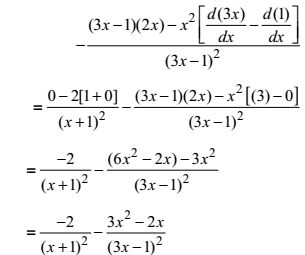10. Find the derivative of cos x from first principle.

f (x) = cos x By first Principle,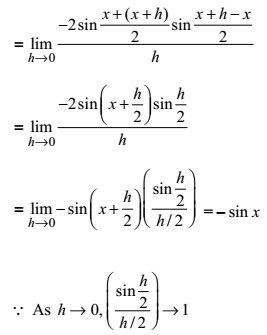11. Find the derivative of the following functions:
(i) sin x cos x
(ii) sec x
(iii) 5 sec x + 4 cos x
(iv) cosec x
(v) 3 cot x + 5 cosec x
(vi) 5 sin x – 6 cos x + 7
(vii) 2tanx – 7 sec x

(i) Let f(x) = sinx cosx
f’(x) = u’v + uv’
= (d/dx sin x) cosx + sinx d/dx (cos x)
= cos x. cos x + sin x(-sin x)
cos2x – sin2x = cos2x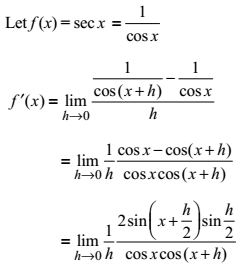(ii)(iii) Let f(x) = 5secx + 4cos x
f’(x) = (d(5sec x + 4cos x))/dx
= (d(5sec x)/dx) + (d(4cosx)/dx)
= 5.(d(sec x)/dx) + 4.(d(cos x)/dx)
= 5sec x tan x – 4 sin x
(iv) Let f(x) = cosec x
f'(x) = (d(cosec x))/dx = (d. 1/sinx)/dx
= (sinx (d(1)/dx) – (1.d(sin x)/dx))/(sin x)2
= (0 – cos x)/(sin 2 x) = (-cosx/sin x). (1/sin x)
= - cosec x cot x
(v) Now,
d/dx (3 cot x) = 3. d/dx (cot x) = -3 cosec2 x
Also, 5.d/dx (cosec x) = - 5cosec x cot x
d/dx (3cot x + 5 cosec x)
= -3 cosec2x – 5cosec x cot x
= -cosec x (3cosec x + 5 cot x)
(vi) Let f(x) = 5 sin x – 6 cos x + 7
f'(x) = (d(5 sin x – 6 cos x + 7))/dx
= (d(5 sin x))/dx - (d(6 cos x))/dx + (d(7))/dx
= 5cos x – 6(- sin x) + 0
= 5 cos x + 6 sin x